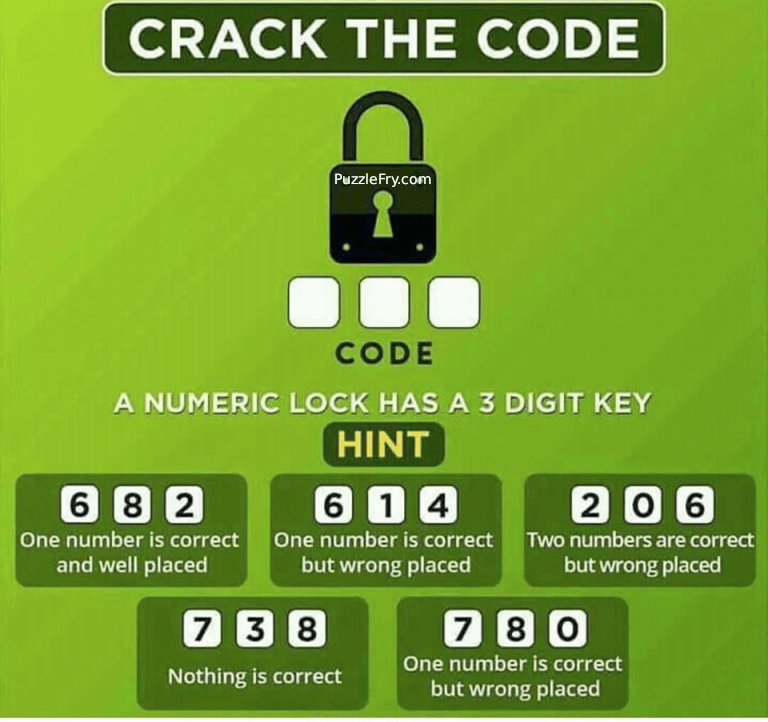# Crack the code puzzle

190.4K Views# A numeric lock has a 3 digit key-

HINT-

A. 6 8 2 – One Number is correct and well placed
B. 6 1 4 – One Number is correct but wrong place
C. 2 0 6 – Two Numbers are correct but Wrong Places
D. 7 3 8 – Nothing is correct
E. 8 7 0 – One Number is correct but wrong place

## The code is 0 4 2

Explanation-
A. 6 8 2 – One Number is correct and well placed – Number cannot be 6 as that will make Statement B wrong so the number in code is 2 which is correctly placed also(on Position 3)
B. 6 1 4 – One Number is the correct but wrong place – 6 cannot be that number as per Statement A, It cannot be 1 also as if the number is 1 it can be on either on 1st or 3rd place those are already taken by digits 0 and 2 so the only number left is 4
C. 2 0 6 – Two Numbers are correct but Wrong Places – One of digit which is correct is 0 as per Statement E but its place cannot be at second(as per statement C) and third position( as per Statement E) so it can be only one first place, another digit is 2 as per statement A.
D. 7 3 8 – Nothing is correct – We can rule out these 3 digits from other statements
E. 8 7 0 – One Number is the correct but wrong place – We know 0 is one of the digit in code but the place for it would be not 3

From statements A, B and E we got our numbers.
Statement A gave a position for Number 2. – Statement A -> _ _ 2
Statement C and E gave a position for the other 2 numbers. Statement A, C and E-> 0 _ 2
Statement B -> last digit 4 which will come in the middle so code would be 0 4 2

Finally, the code is 0 4 2

The code is 102. It fits all the conditions.